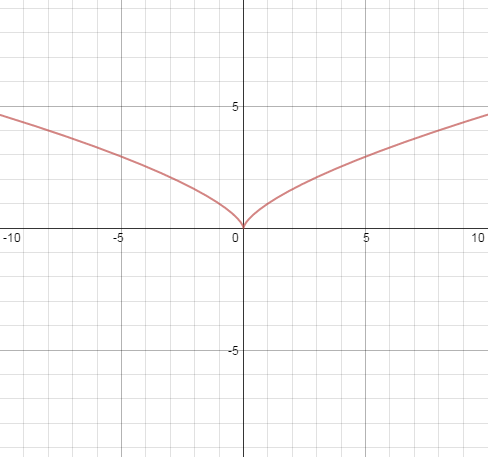User Name Remember Me? Password

 Calculus Calculus Math Forum

 March 21st, 2016, 07:55 PM #1 Member   Joined: Mar 2015 From: Los Angeles Posts: 73 Thanks: 7 critical numbers and local extrema I'm learning calculus along with a student I'm tutoring. I have a few questions about critical numbers and local extrema. As I understand it, a "critical number" is a value of the independent variable in which the first derivative either equals zero or is undefined, AND the function is defined at that number. Is this a strict definition, 100% complete and correct? The term "critical number" is distinguished from "critical point"---the latter refers to the point $(x, f(x) )$ So if the definition is complete, that means, for example, there is no such thing as a critical number $x$ at which $f(x)$ is undefined. Is that true? Then the book gives the following procedure for finding local extrema. (It also uses the term relative extrema rather than local extrema.) Find all critical numbers $x_1, x_2, x_3, ...$ Make a list of intervals $(- \infty, x_1), (x_1, x_2), (x_2, x_3), (x_3,\infty)$. (Adjust this list if the domain of the $f$ is not all real numbers.) Pick a point inside each interval and evaluate $f'(x)$. Note if it's positive or negative. Look at each critical number $c$. If the sign of $f'(x)$ changes from negative to positive at $c$, then $(c,f(c))$ is a local minimum. Vice-versa, it's a local maximum. If the sign does not change, then there's no extremum at c. Is this all 100% correct? Are there any exceptions to this procedure? As an example of a function that has a place where $f'(0)$ is undefined (but $f(0)$ is defined), meaning $0$ is a critical number, is $f(x) = x^{2 \over 3}$. I love this function. Consider the generic power function $f(x) = x^a$. For what values of $a$ does this result in a first derivative that is undefined at zero? I believe that would be $a < 1, a \neq 0$. What if we pick $a = {1 \over 2}$ ? That would be a function defined at 0, while its first derivative is not. But neither $f(x)$ nor $f'(x)$ would be defined for negative x, meaning there's no local extrema at 0 (depending on how you define local extrema). What if we pick $a = {1 \over 3}$ ? This is defined on all x because of the interesting property that the cube root can be found for negative numbers, while its first derivative is not defined at 0. But again there's no local extrema at 0 So if we pick $a= {2 \over 3}$ it's perfect! We have a local minimum! Plus it demonstrates a critical number that results from $f'(0)$ being undefined. Here's a plot:March 21st, 2016, 10:27 PM   #2
Global Moderator

Joined: Dec 2006

Posts: 21,026
Thanks: 2257

Quote:
 Originally Posted by mike1127. . . a "critical number" is a value of the independent variable in which the first derivative either equals zero or is undefined, AND the function is defined at that number. Is this a strict definition, 100% complete and correct?
That's clearly not 100% complete and correct. It doesn't make sense to refer to the first derivative unless the function is differentiable, but the definition you gave doesn't specify that the function is differentiable. The usual term is "critical value" rather than "critical number".March 22nd, 2016, 12:17 AM #3 Member   Joined: Mar 2015 From: Los Angeles Posts: 73 Thanks: 7 Thanks, that's a very helpful point! The book I'm using is called "Calculus for the Life Sciences" and honestly it's calculus-lite. I am not sure it's complete or exact about most things. I will use "critical value" from now on, not "critical number" (even though the book uses the latter). So what would be a more useful formulation of the procedure for finding local extrema? Maybe something like this? Start with an interval $(a,b)$ on which $f(x)$ is defined, and $f'(x)$ is defined everywhere except for a finite number of points. Choose critical values by solving for $f'(x) = 0$ and also including values for which $f'(x)$ is undefined. Then find the sign of $f'$ between each critical value, and observe each $x_i$ where it changes sign. Then $(x_i, f(x_i))$ will be a local extrema. Something like that? But what do we have to consider about the endpoints of $(a,b)$? How do we choose $(a,b)$ anyway? In this calculus text, most problems have some practical bent and there is a natural interval of interest. What if we asked, "Where are ALL the local extrema?" Do we have to determine the domain of $f$, which may include discontiguous intervals, and then apply the procedure above for each interval? But clearly this kind of thing will lead to a mess for, say, a step function. I know I'm asking scattershot questions here, so let me try to clarify my intentions. I studied calculus a long time ago, and I wish to get good at tutoring AP calculus. For now I am tutoring a student who is working out of the Life Sciences book, so it's not ideal, but maybe my question should be: what would AP calculus students be expected to know about using the derivative to find local extrema?Tags critical, extrema, local, numbersThread ToolsShow Printable VersionEmail this Page Display ModesLinear ModeSwitch to Hybrid ModeSwitch to Threaded ModeSimilar Threads Thread Thread Starter Forum Replies Last Post CPAspire Pre-Calculus 2 March 27th, 2015 07:52 AM crnogorac Calculus 1 December 24th, 2013 04:03 AM ckooiker Calculus 2 November 5th, 2011 10:29 AM Calc12 Calculus 9 December 14th, 2010 08:18 PM lovetolearn Algebra 0 December 31st, 1969 04:00 PM

 Contact - Home - Forums - Cryptocurrency Forum - Top